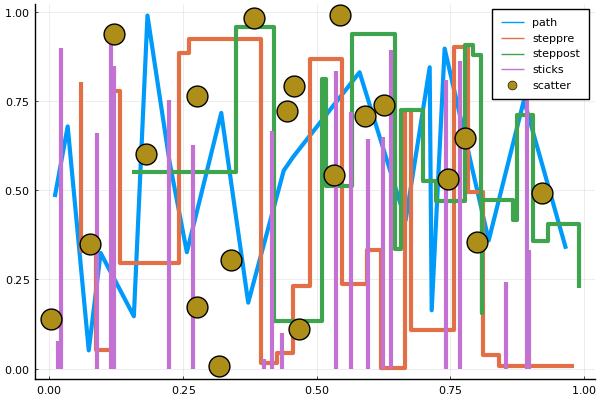# Line typesusing Plots
pyplot()
linetypes = [:path :steppre :steppost :sticks :scatter]
n = length(linetypes)
x = Vector[sort(rand(20)) for i = 1:n]
y = rand(20, n)
plot(x, y, line = (linetypes, 3), lab = map(string, linetypes), ms = 15)